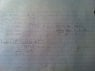# Taylor Polynomial with Remainder Question

• JustinLiang
In summary, the minimal degree Taylor polynomial you need to calculate sin(1) to 3 decimal places is 6 decimal places. However, an easier way is to start computing the terms of sin(1) one-by-one, and noting that you have an alternating series. What do you know about the "truncation" (remainder) error in an alternating series?f

## Homework Statement

What is the minimal degree Taylor polynomial about x=0 that you need to calculate sin(1) to 3 decimal places? 6 decimal places?

## Homework Equations

R_nx = f^(n+1)(c)(x-a)^(n+1)/(n+1)(factorial)

## The Attempt at a Solution

I have attached my attempt. I am stuck on the last step, how do I solve for n? Did I even do it right up until now?

#### Attachments

•photo (5).jpg
27.5 KB · Views: 986

## Homework Statement

What is the minimal degree Taylor polynomial about x=0 that you need to calculate sin(1) to 3 decimal places? 6 decimal places?

## Homework Equations

R_nx = f^(n+1)(c)(x-a)^(n+1)/(n+1)(factorial)

## The Attempt at a Solution

I have attached my attempt. I am stuck on the last step, how do I solve for n? Did I even do it right up until now?

A MUCH easier way is to start computing the terms of sin(1) one-by-one, and noting that you have an alternating series. What do you know about the "truncation" (remainder) error in an alternating series?

RGV

A MUCH easier way is to start computing the terms of sin(1) one-by-one, and noting that you have an alternating series. What do you know about the "truncation" (remainder) error in an alternating series?

RGV

But don't you need a calculator for that? You would have to calculate sin1 and compare your approximations to see the difference (remainder).

But don't you need a calculator for that? You would have to calculate sin1 and compare your approximations to see the difference (remainder).

No, you don't need to know the value of sin(1)---remember, sin(1) is the thing that you are trying to compute!

RGV

No, you don't need to know the value of sin(1)---remember, sin(1) is the thing that you are trying to compute!

RGV

Wow, I totally misread the question... Ok so now I have

Pn(x) = x - x^3/3! + x^5/5! - x^7/7! + x^9/9! - x^11/11! + ...
Pn(1) = 1 - 1/6 + 1/120 - 1/5040 + 1/362880

How do I know which is 3 decimal and 6 decimal places without a calculator?

Wow, I totally misread the question... Ok so now I have

Pn(x) = x - x^3/3! + x^5/5! - x^7/7! + x^9/9! - x^11/11! + ...
Pn(1) = 1 - 1/6 + 1/120 - 1/5040 + 1/362880

How do I know which is 3 decimal and 6 decimal places without a calculator?

Are you not allowed to use a calculator to do simple addition, subtraction and division? if not, then welcome to the world of manual computation from 50 years ago: this CAN be done by hand, but it is unpleasant.

RGV

Are you not allowed to use a calculator to do simple addition, subtraction and division? if not, then welcome to the world of manual computation from 50 years ago: this CAN be done by hand, but it is unpleasant.

RGV

Haha, our prof said we don't need a calculator for his course. But it seems like we do for the assignments.

Back to the question... I am still somewhat clueless. First off, when they said 3 decimals places, would that mean <10^-2? It seems like the 7th derivative at 1/5040 would be a plausible answer but the answer key says 6... What do I do :S

Haha, our prof said we don't need a calculator for his course. But it seems like we do for the assignments.

Back to the question... I am still somewhat clueless. First off, when they said 3 decimals places, would that mean <10^-2? It seems like the 7th derivative at 1/5040 would be a plausible answer but the answer key says 6... What do I do :S

No. Three-decimal places of accuracy require an |error| < 0.5*10^-4 = 1/2000, so stopping at the term -1/5040 will do (but be sure to INCLUDE that term). Six decimals of accuracy need an |error| < 0.5x10^-7 = 1/20,000,000, so you can figure out where you have to stop the series.

RGV

Haha, our prof said we don't need a calculator for his course. But it seems like we do for the assignments.

Back to the question... I am still somewhat clueless. First off, when they said 3 decimals places, would that mean <10^-2? It seems like the 7th derivative at 1/5040 would be a plausible answer but the answer key says 6... What do I do :S

No. Three-decimal places of accuracy require an |error| < 0.5*10^-4 = 1/20,000, so stopping at the term -1/362,880 will do (but be sure to INCLUDE that term). Six decimals of accuracy need an |error| < 0.5x10^-7 = 1/20,000,000, so you can figure out where you have to stop the series.

RGV